# Vector Calculus

Many quantities which are of interest in physics are both directed quantities (vectors) and can take on a continuous range of values, making calculus methods necessary. Several operations from the mathematical field of vector calculus are of particular importance in solving physical problems.

 Basic vector calculus operations Vector calculus identities Divergence theorem Stokes' theorem

Index

Vector concepts

 HyperPhysics*****HyperMath*****Calculus R Nave
Go Back

# Vector Calculus Operations

Three vector calculus operations which find many applications in physics are:

1. The divergence of a vector function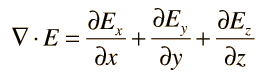2. The curl of a vector function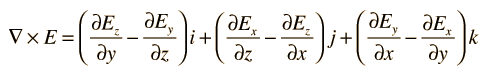3. The Gradient of a scalar function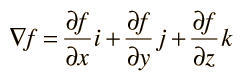These examples of vector calculus operations are expressed in Cartesian coordinates, but they can be expressed in terms of any orthogonal coordinate system, aiding in the solution of physical problems which have other than rectangular symmetries.
Index

Vector calculus

 HyperPhysics*****HyperMath*****Calculus R Nave
Go Back

# The Del Operator

The collection of partial derivative operators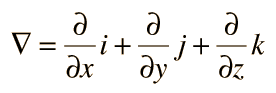is commonly called the del operator. It shows up in important vector calculus operations.

 Gradient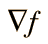Divergence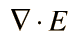CurlLaPlacianIndex

Vector calculus

 HyperPhysics*****HyperMath*****Calculus R Nave
Go Back